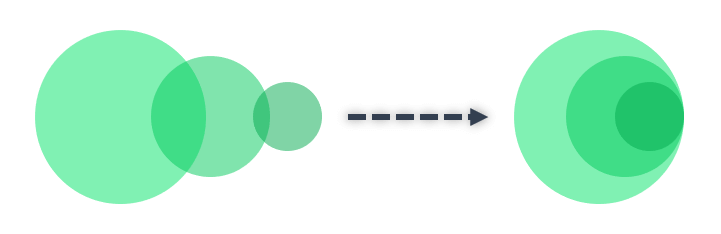# Venn Diagram Template

4.0 (1 review)

Venn Diagram Template will create most used eight dynamic models for showcasing relations between sets. 2 & 3 & 4-circle sets of data in Excel!

TEMPLATE DETAILS
Works on: Windows / Mac (Min. Excel 2007)

PRODUCT DESCRIPTION

Venn diagrams are a widely used method of visualizing how different sets of data relate to one another. The model was first created by a person called John Venn who visualized data in two intersecting circles, hence the naming of the model. However, different models of data sampling and comparing have been developed by other mathematicians and statisticians ever since. In Venn Diagram Template, we have created dynamic models of the eight most used models which will cover almost all of your needs for showcasing relations between sets.

The models cover ranges from two to four sets of data in Excel within more manageable sections.

##Venn Diagram Template Features:

Venn Diagram Template consists of 8 models:

• 2 Circles Model
• Triangular Model
• 3 Circles Linear Model
• 3 Circles Stacked Model
• Square Model
• 4 Circles Linear Model
• Flower Model
• 4 Circles Stacked Model

### 2 Circles Model

The first model is very basic two circle diagram, which helps users compare two data sets and how they relate to one another. This model can be used for all kinds of data, as you are free to change both titles and content of the columns. The diagram is dynamic and will update automatically.

### Triangular Model

The second model is a very common triangular Venn diagram. This model allows users to compare three different data sets with five different visualizations: unique data, three possible two-set intersections, and an intersection that shows data from all three sets.### 3 Circles Linear Model

The linear model of the three-circle diagram has ability to show how only two sets from a total of three relate to each other. As the name suggests, you can visualize your data in a linear correlation.### 3 Circles Stacked Model

The next model in Venn Diagram Template is a stacked model of 3 circles, which is best used to describe how two smaller sets belong to a larger set. You can also use this model to demonstrate a relation that spreads from a “nucleus” or central idea.### Square Model

The square model will help you visualize the relation between four sets, with four unique data visualization, four two-way intersections, four three-way intersections and one intersection that shows data from all sets. Please note that this model will not visualize intersections between columns 1 & 4 or 2 & 3 and you will see a warning for not supported intersections.If you want to visualize such data, please use the following flower model.

### 4 Circles Linear Model

Another model of showing relations between four data sets is the linear four-circle diagram. It is similar to the 3-circle model and you can use this one to show how set pairs from a total of four relate to each other in a linear method.

### Flower Model

The most inclusive model of Venn Diagram Maker that we have created is the flower model. This diagram makes it possible for you to visualize all kinds of relations between four data sets with no restrictions.

### 4 Circles Stacked Model

The final model of our template is a stacked diagram with four circles. The idea is similar to the 3-circle stacked model, but this one visualizes not two but three categories that belong to a larger idea or category.Please note that everything in this template is dynamic. So you only need to input your data in the tables and the diagrams.

## Venn Diagram Template Features Summary:

• Dynamic Venn Diagram Maker in Excel
• Easy to fill 8 different Venn Diagram Models
• No installation needed.
• Compatible with Excel 2007 and later versions
• Does not include any VBA macros
• Works on both Mac and Windows
• On-sheet instructions

## 1 review for Venn Diagram Template

1. Chris Mody

The design seems great. Suitable for microsoft excel users but it is not working properly in google sheets.

• Someka

Hello, Chris